# Chapter 5.1. Loops

In this chapter, we will become familiar with how to repeat blocks of commands, known in programming as "loops". We will write a number of loops using the for operator in its classic form. Finally, we will solve some practical problems, which require repeating a series of actions, using loops.

## Repeating Blocks of Code (For Loop)

In programming, it is often required to execute a block with commands multiple times. For this reason, we are using loops. We can see an example of a for loop, which goes through the numbers from 1 to 10 and prints them: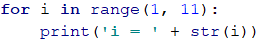The loop starts with the operator for and passes through all values of a particular variable in a given range, for example, all numbers from 1 to 10 included (without 11), and for each value, it performs a series of commands.

Upon declaring the loop, you can specify a start value and end value, while the end value is not included in the range. The body of the loop is a block with at least one command. The figure below shows the structure of a for loop:In most cases, a for loop is run between 1 and n (for example from 1 to 10). The purpose of the loop is to go sequentially through the numbers 1, 2, 3, …, n and for each of them to perform a particular action. In the example above, the variable i accepts values from 1 to 10 and the current value is printed in the body of the loop. The loop is repeated 10 times and each of these repetitions is called "iteration".

### Problem: Numbers from 1 to 100

Write a program that prints the numbers from 1 to 100. The program does not accept input and prints the numbers from 1 to 100 sequentially, each on a separate line.

#### Hints and Guidelines

We can solve the problem using a for loop, via which we will pass through the numbers from 1 to 100 using the i variable, and print the numbers in the body of the loop:Start the program with [Ctrl+Shift+F10] or with right-click - Run and test it:#### Testing in The Judge System

You should get 100 points (fully accurate solution).

### Problem: Numbers Ending in 7

Write a program that finds all numbers in the range [1 … 1000], that end in 7.

#### Hints and Guidelines

We can solve the problem by combining a for loop for passing through the numbers between 1 and 1000 and a condition to check if each of the numbers ends in 7. Of course, there are other solutions, but let's solve the problem using loop + condition: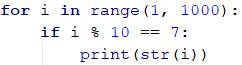### Problem: Latin Letters

Write a program that prints the letters from the Latin alphabet: a, b, c, …, z.

#### Hints and Guidelines

It is good to know that the for loops don't work only with numbers. We can solve the task by running a for loop, that goes sequentially through all letters in the Latin alphabet:### Problem: Sum Numbers

Write a program that reads n integers and finds their sum.

• The first line of the input holds the number of integers n.
• Each of the following n holds an integer.
• Sum up the numbers and print the result.

Input Output
2
10
20
30
3
-10
-20
-30
-60
4
45
-20
7
11
43
1
999
999
0 0

#### Hints and Guidelines

We can solve the problem by summing up numbers in the following way:

• We read the input number n.
• We initially start with a sum sum = 0.
• We run a loop from 0 to n. On each step of the loop, we read the number num and add it to the sum.
• Finally, we print the calculated amount sum.

Here is the source code for the solution: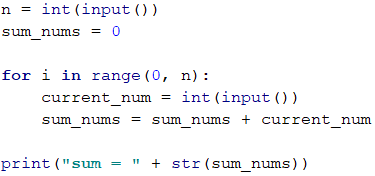### Problem: Max Number

Write a program that enters n integers (n > 0) and finds the max among them. The first line of the input reads the number of integers. After that, the next lines read the integers, each on a separate line. Examples:

Input Output
2
100
99
100
3
-10
20
-30
20
4
45
-20
7
99
99
1
999
999
2
-1
-2
-1

#### Hints and Guidelines

We will first enter one number n (the number of integers that are about to be entered). We assign the current maximum maxan initial neutral value, for example -1000000. Using a for loop, that is iterated n-1 times, we read one integer num. If the read number num is higher than the current maximum max, we assign the value of the num to the max value. Finally, in max, we must have stored the highest number. We print the number on the console.: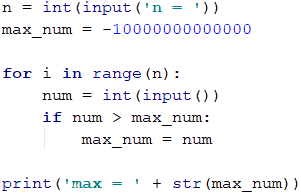### Problem: Min Number

Write a program that enters n integers (n > 0) and finds the min among them. The first line of the input reads the number of integers and after that, the next lines read the integers, each on a separate line.

Input Output
2
100
99
99
3
-10
20
-30
-30
4
45
-20
7
99
-20

#### Hints and Guidelines

The problem is completely identical to the previous one, except this time we will start with another neutral starting value: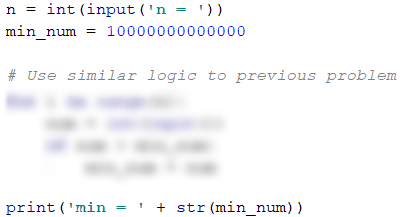### Problem: Left and Right Sum

Write a program that reads an input 2 * n integers and checks if the sum of the first n numbers (left sum) is equal to the sum of the second n numbers (right sum). In case the sums are equal, print "Yes" + sum, otherwise print "No" + the difference. The difference is calculated as a positive number (by absolute value). The format of the output must be identical to the one in the examples below.

#### Sample Input and Output

Input Output Input Output
2
10
90
60
40
Yes, sum = 100 2
90
9
50
50
No, diff = 1

#### Hints and Guidelines

We will first input the number n, after that the first n numbers (left half) and we sum them up. We will then proceed with inputting more n numbers (right half) and sum them up. We calculate the difference between the sums by absolute value: math.fabs(rightSum - leftSum). If the difference is 0, print "Yes" + sum, otherwise print "No" + the difference .### Problem: Odd Even Sum

Write a program that inputs n integers and checks whether the sum of the numbers on even positions is equal to the sum of the numbers on odd positions. In case the sums are equal, print "Yes" + sum, otherwise print "No" + the difference . The difference is calculated by absolute value. The format of the output must be identical to the one in the examples below.

Input Output
4
10
50
60
20
Yes
Sum = 70
4
3
5
1
-2
No
Diff = 1
3
5
8
1
No
Diff = 2

#### Hints and Guidelines

Input the numbers one by one and calculate the two sums (of the numbers on even positions and the numbers on odd positions). Identically to the previous problem, we calculate the absolute value of the difference and print the result ("Yes" + sum in case of difference of 0 or "No" + the difference in any other case):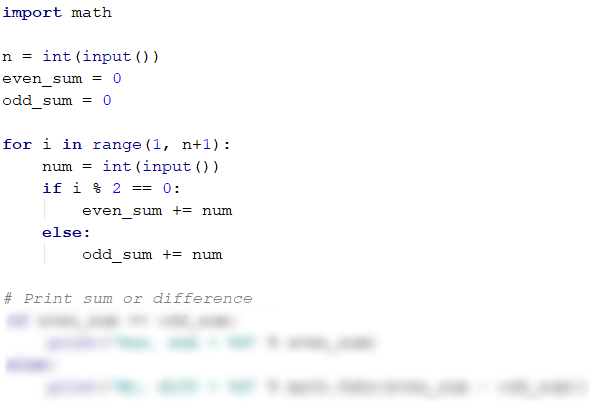### Problem: Vowels Sum

Write a program that inputs text (string), calculates and prints the sum of the values of vowels according to the table below:

a e i o u
1 2 3 4 5

#### Sample Input and Output

Input Output Input Output
hello 6
(e+o = 2+4 = 6)
bamboo 9
(a+o+o = 1+4+4 = 9)
hi 3
(i = 3)
beer 4
(e+e = 2+2 = 4)

#### Hints and Guidelines

We read the input text line, null the sum, and run a loop, which goes through each symbol from the text. We check each letter c and verify if it is a vowel, and accordingly, add its value to the sum:## What Have We Learned from This Chapter?

We can repeat a block of code with a for loop:We can read a sequence of n numbers from the console:## Problems: Loops

Now, that we've become acquainted with the loops, it's time to consolidate our knowledge in practice, and as you know, -> this is done through writing lots of code. Let's solve a few problems for exercise.

### Creating New Project in PyCharm

Create a new project in PyCharm (from [File] -> [New Project]), to organize better the exercise tasks. The purpose of this project is to contain one Python file for each task from the exercises: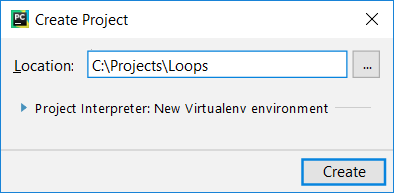### Problem: Half Sum Element

Write a program that inputs n integers and checks whether there is a number among them, which is equal to the sum of all the rest. If there is such an element, print "Yes" + its value, otherwise print - "No" + the difference between the largest element and the sum of the rest (by absolute value).

#### Sample Input and Output

Input Output Comment
7
3
4
1
1
2
12
1
Yes
Sum = 12
3 + 4 + 1 + 2 + 1 + 1 = 12
4
6
1
2
3
Yes
Sum = 6
1 + 2 + 3 = 6
3
1
1
10
No
Diff = 8
|10 - (1 + 1)| = 8
3
5
5
1
No
Diff = 1
|5 - (5 + 1)| = 1
3
1
1
1
No
Diff = 1
-

#### Hints and Guidelines

We have to calculate the sum of all elements, find the largest of them, and check the condition.

### Problem: Odd \/ Even Positions

Write a program that reads n numbers and calculates the sum, the min, and max values of the numbers on even and odd positions (counted from 1). If there are no min / max elements, print "No".

#### Sample Input and Output

Input Output Input Output
6
2
3
5
4
2
1
OddSum=9,
OddMin=2,
OddMax=5,
EvenSum=8,
EvenMin=1,
EvenMax=4
2
1.5
-2.5
OddSum=1.5,
OddMin=1.5,
OddMax=1.5,
EvenSum=-2.5,
EvenMin=-2.5,
EvenMax=-2.5
1
1
OddSum=1,
OddMin=1,
OddMax=1,
EvenSum=0,
EvenMin=No,
EvenMax=No
0 OddSum=0,
OddMin=No,
OddMax=No,
EvenSum=0,
EvenMin=No,
EvenMax=No
5
3
-2
8
11
-3
OddSum=8,
OddMin=-3,
OddMax=8,
EvenSum=9,
EvenMin=-2,
EvenMax=11
4
1.5
1.75
1.5
1.75
OddSum=3,
OddMin=1.5,
OddMax=1.5,
EvenSum=3.5,
EvenMin=1.75,
EvenMax=1.75
1
-5
OddSum=-5,
OddMin=-5,
OddMax=-5,
EvenSum=0,
EvenMin=No,
EvenMax=No
3
-1
-2
-3
OddSum=-4,
OddMin=-3,
OddMax=-1,
EvenSum=-2,
EvenMin=-2,
EvenMax=-2

#### Hints and Guidelines

This task combines some of the previous tasks: finding the min, max value, and sum, as well as processing of elements on even and odd positions . Check them out.

In the current task, it is better to work with fractions (not integers). The sum, the min, and the max will also be fractions. We must use a neutral starting value when finding the min / max value, for example 10000.0 and -10000.0. If the result is the neutral value, print "No".

### Problem: Equal Pairs

There are 2 * n numbers. The first and the second number form a pair, the third and the fourth number as well, and so on. Each pair has a value – the sum of its numbers. Write a program that checks if all pairs have equal value. In case the value is the same, print "Yes, value=…" + the value, otherwise print the maximum difference between two neighboring pairs in the following format - "No, maxdiff=…" + the maximum difference. The input consists of the number n, followed by 2*n integers, all of them one per line.

#### Sample Input and Output

Input Output Comment
3
1
2
0
3
4
-1
Yes, value=3 values = {3, 3, 3}
equal values
2
1
2
2
2
No, maxdiff=1 values = {3, 4}
differece = {1}
max difference = 1
4
1
1
3
1
2
2
0
0
No, maxdiff=4 values = {2, 4, 4, 0}
differences = {2, 0, 4}
max difference = 4
1
5
5
Yes, value=10 value = {10}
one vlaue
equal values
2
-1
0
0
-1
Yes, value=-1 values = {-1, -1}
equal values
2
-1
2
0
-1
No, maxdiff=2 values = {1, -1}
differences = {2}
max difference = 2

#### Hints and Guidelines

We read the input numbers in pairs. For each pair, we calculate its sum. While reading the input pairs, for each pair except the first one, we must calculate the difference compared to the previous one. To do that, we need to store as a separate variable the sum of the previous pair. Finally, we find the largest difference between two pairs. If it is 0, print "Yes" + the value, otherwise print - "No" + the difference.

## Lab: Graphics and Web Applications

In the current chapter, we learned about loops as a programming construction that allows repeating a particular action or a group of actions multiple times. Now let's play with them. To do that, we will draw some figures that will consist of a large number made up of repeating graphical elements, but this time we will not do it on the console, but in a graphical environment using "turtle graphics". It will be interesting. And it is not hard at all. Try it!

### Problem: Turtle Graphics GUI Application

The purpose of the following exercise is to play with a drawing library, also known as "turtle graphics". We will build a graphical application in which we will draw various figures, by moving our "turtle" across the screen via operations like "move 100 positions ahead", "turn 30 degrees to the right", "move 50 more positions ahead". The application will look approximately like this: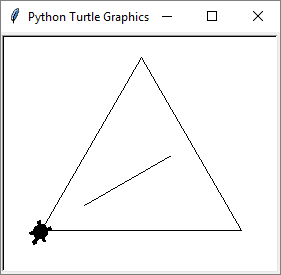Let's first get familiar with the concept of drawing "Turtle Graphics". Take a look at the following sources:

We will start by creating a new project in PyCharm: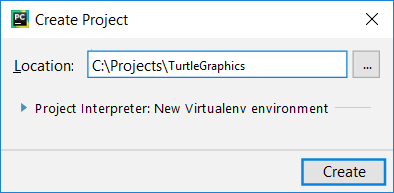In the newly created project, we add a new Python File. For the drawing, we will use the external library turtle. It defines class Turtle, which represents drawing turtle. To use it, we add the following code at the beginning of the Python file: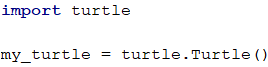After that for the drawing we add the code:The above code moves and rotates the turtle, which is located in the center of the screen at the beginning (in the middle of the form) and draws an equilateral triangle. You can edit it and play with it. Start the app: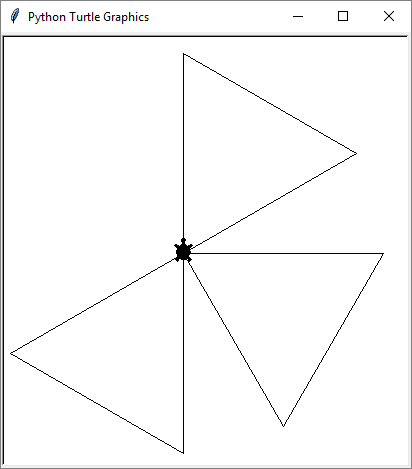Now you can modify the turtle code and make it more complex, for the turtle to make a more complex figure: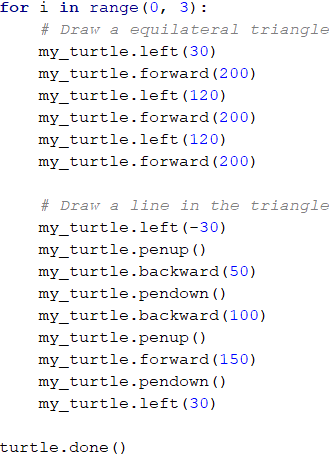Again start the app to see the result: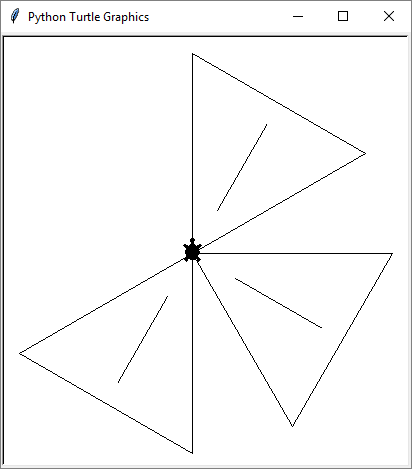Now our turtle draws more complex figures via a nice animated motion.

### Problem: * Draw a Hexagon with The Turtle

Add a new Python file, with the name hexagon, which will draw a hexagon: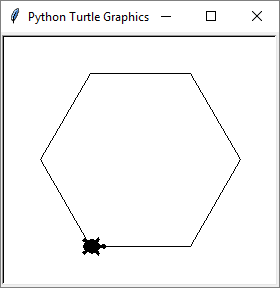Hint:

With loop repeat the following 6 times:

• 60 degrees rotation.
• Forward step of 100.

### Problem: * Draw a Star with The Turtle

Add new Python file star.py, which draws a star with 5 beams (pentagram), as on the figure below: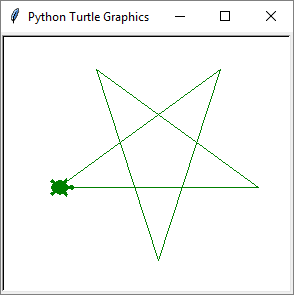Hints: Change the colour: my_turtle.color("green").

Repeat 5 times the following in a loop:

• Forward step of 200.
• 144 degrees rotation.

### Problem" * Draw a Spiral with The Turtle

Add new Python file spiral.py, which draws spiral with 20 beams as on the figure below: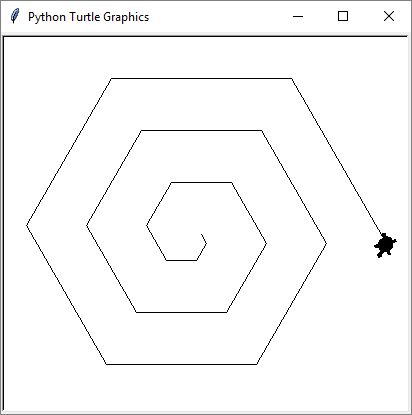Hint: Draw in a loop by moving ahead and rotating. In each step, decrease the length gradually of the forward step and rotate 60 degrees.

### Problem: * Draw a Sun with The Turtle

Add a new Python file sun.py, which draws a sun with 36 beams, as on the figure below: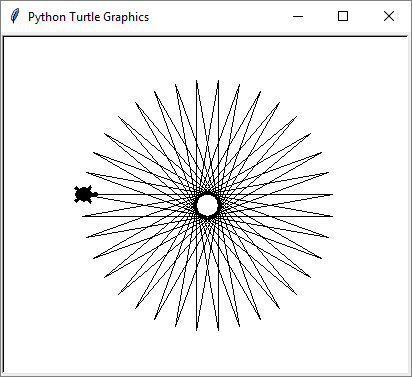### Problem: * Draw a Spiral Triangles with The Turtle

Add new Python file triangle.py, which draws three triangles with 22 beams each, as on the figure below: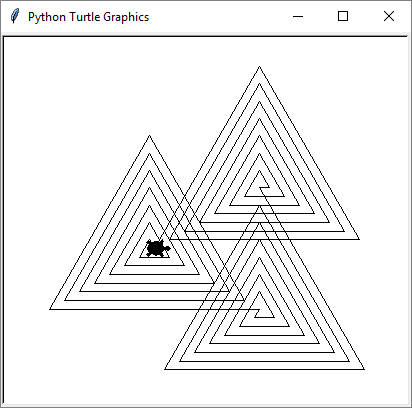Hint: Draw in a loop by moving forward and rotating. In each step, increase the length of the forward step by 10 and rotate 120 degrees. Repeat 3 times for the three triangles.

If you have a problem with the above exercises, ask for help in the SoftUni's Reddit Community: https://www.reddit.com/r/softuni/.